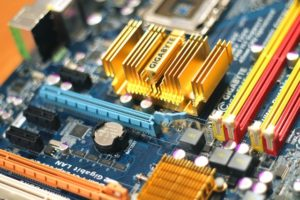A power factor is a unitless number used in alternating current circuits. It is defined as the ratio of the working power used in electrical circuits that are measured in kilowatts. It is the amount of power used to run various machinery and equipment for a certain period of time. An increase and decrease in power factor can be calculated with the help of a power factor calculator. Improving the power factor can maximize the current carrying capacity and reduces the phase differences between voltage and current. It increases the supply of voltage to the equipment and lowers the cost of electricity bills. Lower the power factor, higher is a load of current. Due to the low power factor, large power loss is to be faced as the conductor carries a large current. It also causes high voltage loss in alternators and transmission lines and due to it, the system reduces handling capacity.The reason behind the low power factor is inductive loads. In a purely inductive circuit, the current lags 90 degrees from voltage, and the differences of phase angle become very large between the current and the voltage leading to zero power factor. Some responsible devices for low power factors are the single and three-phase induction motors, Industrial heating furnaces, Electrical discharge lamps, Transformers, Harmonic Currents, and varying load in the power system.

In an industry, almost 90% of the load consists of Induction Machines. Such machines use magnetizing current to make themselves run and hence work at a low power factor. The induction motor runs at a very low power factor at about 0.2 – 0.3, The electric transformers, electric furnaces, generators, arc lamps, etc all work at a very low power factor.

At the present time, the interconnected power system is used. Because of this, the loading conditions of the power system vary with season and time thus results in peak as well as low load periods. A low power factor also happens when the working system is tightly loaded because of which the voltage increases and the current supplied by the machine also increases and the power factor lowers down. A low power factor also occurs when the system processes harmonic current, improper wiring, electrical accidents.

Low power factors can be corrected by using capacitors to draw leading currents, by this the lagging of inductive current can be canceled and hence the power factor can be improved.

Power factor can be improved or can be corrected by using some equipment like Capacitor Bank which is the simplest method applied in the areas of large lagging currents. These banks are star connected or delta connected where a control system is provided that monitors the power factor and switches the capacitors on or off. Capacitor Banks are lightweight and are easy to install, require low maintenance, and no foundation. Capacitor banks work as a source of local reactive power that reduces the phase differences between the voltage and the current. In this process, the power of the voltage and the current is measured using a voltmeter, and the ammeter is calculated with the help of the Power factor calculator.